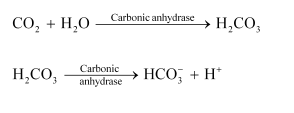# What are the major transport mechanisms for $mathrm{CO}_{2}$ ? Explain.

Question:

What are the major transport mechanisms for $\mathrm{CO}_{2}$ ? Explain.

Solution:

Plasma and red blood cells transport carbon dioxide. This is because they are readily soluble in water.

(1) Through plasma:

About $7 \%$ of $\mathrm{CO}_{2}$ is carried in a dissolved state through plasma. Carbon dioxide combines with water and forms carbonic acid.

$$\mathrm{CO}_{2}+\mathrm{H}_{2} \mathrm{O} \longrightarrow \mathrm{H}_{2} \mathrm{CO}_{3}$$

(Carbonic acid)

Since the process of forming carbonic acid is slow, only a small amount of carbon dioxide is carried this way.

(2) Through RBCs:

About $20-25 \%$ of $\mathrm{CO}_{2}$ is transported by the red blood cells as carbaminohaemoglobin. Carbon dioxide binds to the amino groups on the polypeptide chains of haemoglobin and forms a compound known as carbaminohaemoglobin.

(3) Through sodium bicarbonate:

About $70 \%$ of carbon dioxide is transported as sodium bicarbonate. $\mathrm{As} \mathrm{CO}_{2}$ diffuses into the blood plasma, a large part of it combines with water to form carbonic acid in the presence of the enzyme carbonic anhydrase. Carbonic anhydrase is a zinc enzyme that speeds up the formation of carbonic acid. This carbonic acid dissociates into bicarbonate $\left(\mathrm{HCO}_{3}{ }^{-}\right)$and hydrogen ions $\left(\mathrm{H}^{+}\right)$.